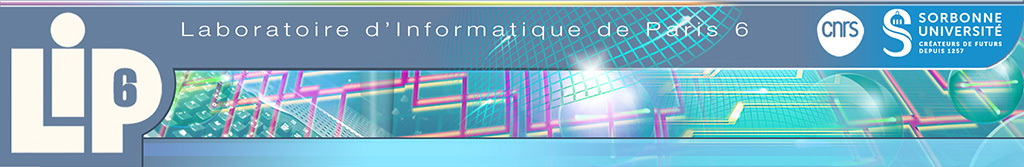#The General Vector Addition System Reachability Problem by Presburger Inductive Invariants

03/09/2010
Relatore/i : Jérôme Leroux (CR CNRS LaBRI)
The reachability problem for Vector Addition Systems (VASs) is a central problem of net theory. The general problem is known decidable by algorithms exclusively based on the classical Kosaraju-Lambert-Mayr-Sacerdote-Tenney decomposition. This decomposition is used in this paper to prove that Parikh images of languages accepted by VASs are semi-pseudo-linear; a class that extends the semi-linear sets, a.k.a. the sets definable in the Presburger arithmetic. We provide an application of this result; we prove that a final configuration is not reachable from an initial one if and only if there exists a Presburger formula denoting a forward inductive invariant that contains the initial configuration but not the final one. Since we can decide if a Preburger formula denotes an inductive invariant, we deduce that there exist checkable certificates of non-reachability. In particular, there exists a simple algorithm for deciding the general VAS reachability problem based on two semi-algorithms. A first one that tries to prove the reachability by enumerating finite sequences of actions and a second one that tries to prove the non-reachability by enumerating Presburger formulas.
BeatriceBerard (at) nulllip6.fr